Advice: Fractional powers of negative numbers

Maple's mathematics is largely based on complex numbers rather than real numbers. In particular, it uses the "principal branch" of fractional powers: the principal branch of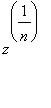has argument between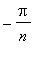and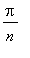, and the argument of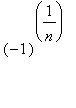is.
So for example:

> evalc((-1)^(1/5));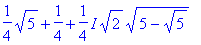> evalf(");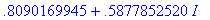> evalf(argument(")/Pi);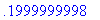The surd function provides "elementary"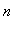'th roots, i.e. if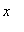is a negative number andis odd, then surd(x,n) is the real (negative)'th root of.

> surd(-8,3);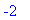In order to have a somewhat nicer syntax, as well as covering rational exponents with numerators other than 1, I have defined an infix-form operator &^ . This is part of the Maple Advisor Database, and must be read in before being used:

> (-1)&^(2/5), (-1) &^ (3/5);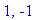For positive,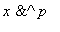is the same as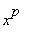. For negative, if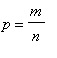is a fraction we have the following cases:

> assume(x<0);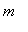andboth odd:> x &^ (3/5);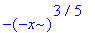even,odd: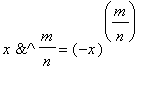> x &^ (4/5);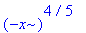odd,even: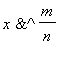is complex.

> x &^ (3/8);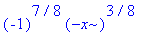Whenis not known to be real, and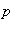is a fraction,is written as a surd. When p is given in decimal form, it is converted to a fraction.

> x:= 'x': x &^ (3/8);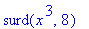> x &^ 0.375;> plot(x &^ (2/3), x = -1 .. 1);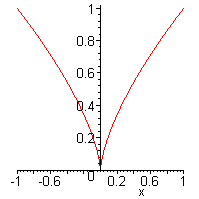> plot(x &^ (3/5), x = -1 .. 1);